# Over fitting in Polynomial Regression

The goal is to understand the concept of over fitting by using polynomial regression.

Over fitting is one of the challenges faced in Machine Learning. It usually occurs when the model that we are trying to implement is too complex or the input data set that we have used for training the model is too small. Due to this the model performs well on the training data set giving us less errors. However, the model suffers when dealing with test data set thus giving large amounts of error.

In our assignment I was tasked with creating 20 pairs of Input data (X,Y) using y=sin(2*pi*x)+N where N stands for Gaussian noise. Then I had to fit polynomial equations of order 0,1,3,9 and plot it to visualize over fitting.

So starting with importing the required libraries and modules

`import numpy as npfrom sklearn.linear_model import LinearRegression, Ridgefrom sklearn.metrics import mean_squared_error, r2_scorefrom sklearn.preprocessing import PolynomialFeaturesimport matplotlib.pylab as pltimport operatorimport pandas as pdfrom scipy.interpolate import make_interp_splinefrom sklearn.model_selection import train_test_splitfrom prettytable import PrettyTable`

Then create 20 pairs of Input data where X has normally distributed values ranging from 0 to 1 and N is random Gaussian noise. Here we use linspace to generate X because linspace gives ordered and evenly spaced out values

`x_data = np.linspace(0, 1, 20).reshape((-1,1))x_nor = np.random.uniform(0, 1, size=20)[:, np.newaxis]N = np.random.normal(scale=0.3 , size=20 )[:, np.newaxis]y_data = np.sin(2*np.pi*x_nor) + N`

Then we split our data set into 10 for Training and 10 for Testing. Here test_size determines how much you want to split the data set. 0.5 means the data set is going to be split in half

`X_Train, X_Test, Y_Train, Y_Test = train_test_split(x_nor, y_data, test_size=0.5)`

Now displaying the data that we would be working with

`print('X: ',x_data)print('Y: ',y_data)`Input Data

Now we create our Linear Regression model initially starting with degree 0

`def LinearReg(x_nor, y_data, Input, degree = 0):    pol_f = PolynomialFeatures(degree = degree)    X_Poly = pol_f.fit_transform(x_nor)    pol_f.fit(X_Poly, y_data)    model = LinearRegression()    fit = model.fit(X_Poly, y_data)    X_plot = pol_f.fit_transform(Input)    y_pred = model.predict(X_plot)        return y_pred, np.array(fit.coef_)`

Now since we have to use polynomial equations of order 0, 1, 3, 9 we need to use the attribute coef_ which assigns weight vectors to the model

`y_pred_0, coef_0 = LinearReg(X_Train, Y_Train, x_data, 0)y_pred_1, coef_1 = LinearReg(X_Train, Y_Train, x_data, 1)y_pred_3, coef_3 = LinearReg(X_Train, Y_Train, x_data, 3)y_pred_9, coef_9 = LinearReg(X_Train, Y_Train, x_data, 9)`

Visualizing the weights in a table format. Using the library PrettyTable that generates ASCII tables for us

`pt =  PrettyTable()pt.field_names = ["W", "M=0","M=1","M=3","M=9"]pt.add_row(["w0",coef_0,coef_1,coef_3,coef_9])pt.add_row(["w1"," " ,coef_1,coef_3,coef_9])pt.add_row(["w2"," " ," ",coef_3,coef_9])pt.add_row(["w3"," " ," ",coef_3,coef_9])pt.add_row(["w4"," " ," "," ",coef_9])pt.add_row(["w5"," " ," "," ",coef_9])pt.add_row(["w6"," " ," "," ",coef_9])pt.add_row(["w7"," " ," "," ",coef_9])pt.add_row(["w8"," " ," "," ",coef_9])pt.add_row(["w9"," " ," "," ",coef_9])print(pt)`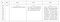Weight Table

We move on to the part of visualizing how the polynomial equations fit our input data.

`def Plot(x,y):    plt1 = plt.gca()    plt1.scatter(X_Train, Y_Train, s=10)    plt1.plot(x, np.sin(2*np.pi*x), color='green')    plt1.plot(x, y, color='red')    plt1.set_ylim((-2, 2))    plt1.set_xlim((0, 1))    plt1.set_ylabel('Y Value')    plt1.set_xlabel('X Value')    plt.show()Plot(x_data,y_pred_0)Plot(x_data,y_pred_1)Plot(x_data,y_pred_3)Plot(x_data,y_pred_9)`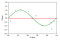Order = 0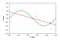Order = 1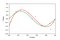Order = 3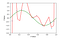Order = 9

We can see that order 0 and 1 are severely under fitting. Order 3 model is fitting model perfectly. Order 9 is getting over fitted as there is a large difference in fitted values. This happens because 20 pairs of data is too small for a 9th order equation to be fit.

So when we visualize the difference in training and test error, we can see that the 9th order train and test errors have a huge difference

`train_err = np.empty(10)test_err = np.empty(10)for degree in range(10):        pol_f= PolynomialFeatures(degree=degree)    x_pol = pol_f.fit_transform(X_Train)    model = LinearRegression()    fit = model.fit(x_pol,Y_Train)        train_err[degree] = mean_squared_error(Y_Train ,model.predict(pol_f.fit_transform(X_Train)))        test_err[degree] = mean_squared_error(Y_Test,model.predict(pol_f.fit_transform(X_Test)))plt.plot(np.arange(10), train_err, color='blue', label="Train Error")plt.plot(np.arange(10), test_err, color='red', label = "Test Error")plt.ylim((0.0, 1e0))plt.ylabel("Error")plt.xlabel("M Value")plt.legend(loc='upper left')`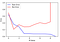Difference in Train and Test Error

To fit our 9th order model, we need to give an input of a much larger data set. So instead of 20 pairs of input values, we generate 100 pairs of input values.

`x_data_2 = np.linspace(0,1,20).reshape((-1,1))x2 = np.random.uniform(0, 1, size =100)[:, np.newaxis]new_N = np.random.normal(scale= 0.3, size = 100)[:, np.newaxis]y2 = np.sin(2*np.pi*x2)+new_N`

Now we create our model again with the new values and try and visualize the data.

`pol_f = PolynomialFeatures(degree=9)x_pol = pol_f.fit_transform(x2)pol_f.fit(x_pol, y2)model = LinearRegression()fit = model.fit(x_pol, y2)x_plot = pol_f.fit_transform(x_data_2)y_pred = model.predict(x_plot)pl1 = plt.gca()pl1.scatter(x2, y2)pl1.plot(x_data_2, np.sin(2*np.pi*x_data_2), color='green')pl1.plot(x_data_2, y_pred, color='red')pl1.set_ylim((-2,2))pl1.set_xlim((0,1))pl1.set_xlabel('Y Data')pl1.set_ylabel('X Data')plt.show()`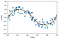9th order model using 100 pairs of input data

We can see that when we increase our input values to 100, the 9th order model is fitting way better than the previous attempt.

To reduce over fitting, a concept called Regularization is used. Regularization works by adding a term to the error function used by the training algorithm. The additional term penalizes large weight values. In our case we use L2 regularization.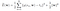L2 Regularization

Here, lambda is the regularization parameter. It is the hyper-parameter whose value is optimized for better results. L2 regularization is also known as weight decay as it forces the weights to decay towards zero (but not exactly zero).

For our data we will need to use a ridge classifier as it takes into consideration the L2 penalty when calculating weights

`alpha = [1, 1e-1, 1e-2, 1e-3, 1e-4, 1e-5]for a in alpha:    pol_f= PolynomialFeatures(degree=9)    x_pol = pol_f.fit_transform(x2)    pol_f.fit(x_pol,y2)    model = Ridge(alpha=a)    fit = model.fit(x_pol,y2)    x_plot = pol_f.fit_transform(x_data_2)    y_pred = model.predict(x_plot)    plt3 = plt.gca()plt3.scatter(x2, y2)    plt3.plot(x_data_2, np.sin(2*np.pi*x_data_2), color='green')    plt3.plot(x_data_2, y_pred, color='r',label="ln lamda = "+str(np.around(np.log(a))))    plt3.set_ylim((-2, 2))    plt3.set_xlim((0, 1))    plt3.set_ylabel('Y Data')    plt3.set_xlabel('X Data')    plt3.legend(loc='upper left')        plt.show()`

Visualizing the training and testing error using regularization

`x2_train, x2_test, y2_train, y2_test = train_test_split(x2, y2, test_size=0.5)train_error_lambda = []test_error_lambda = []alphas = [1.0, 1e-1, 1e-2,  1e-3, 1e-4, 1e-5, 1e-6, 1e-7, 1e-8, 1e-9, 1e-10, 1e-11, 1e-12, 1e-13, 1e-14, 1e-15, 1e-16 ]for alpha in alphas:    pol_f= PolynomialFeatures(degree=9)    x_pol = pol_f.fit_transform(x2_train)    model = Ridge(alpha=alpha)    fit = model.fit(x_pol,y2_train)        train_error_lambda.append(mean_squared_error(y2_train ,model.predict(pol_f.fit_transform(x2_train))) )         test_error_lambda.append(mean_squared_error(y2_test,model.predict(pol_f.fit_transform(x2_test))))plt.plot(np.log(alphas), train_error_lambda, color='green', label='Train Error')plt.plot(np.log(alphas), test_error_lambda, color='red', label='Test Error')plt.ylabel('Errors')plt.xlabel('Lambda Values')plt.legend(loc='upper left')`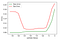Train and Test Error using L2 regularization

## Best performing model

Based on our test, when using L2 regularization, the model with lambda value -20 gave us the least amount of errors. When considering models without regularization, the model with M order = 1 gave us the least amount of errors.

## Challenges faced

1. Plotting the input data onto the graph was an issue. After gaining some guidance, learnt that we need to reshape the array to plot it.
2. At first I tried to use the input values in a non-ordered fashion. This would not plot well as random points would be made on the graph. This was overcome by using the concept of linspace which basically gives us an ordered and evenly spaced values when taking as input.
3. When trying to fit model with order = 0, the program would throw an error and not fit the model. This was overcome by calling the LinearRegression API and setting the initial degree to 0. For later orders, I used the coef_ attribute.

## References

Software Engineer in the making

## More from Shonit Gangoly

Software Engineer in the making